# Cox regression model

(diff) ← Older revision | Latest revision (diff) | Newer revision → (diff)

A regression model introduced by D.R. Cox [a4] and subsequently proved to be one of the most useful and versatile statistical models, in particular with regards to applications in survival analysis (cf. also Regression analysis).

Letbe stochastically independent, strictly positive random variables (cf. also Random variable), to be thought of as the failure times ofdifferent items, such thathas hazard function(i.e.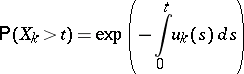for) of the form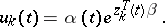Here,is an unknown hazard function, the baseline hazard obtained if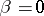, and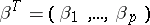is a vector ofunknown regression parameters. Thedenote known non-random vectors of possibly time-dependent covariates, e.g. individual characteristics of a patient referring to age, sex, method of treatment as well as physiological and other measurements.

The parameter vectoris estimated by maximizing the partial likelihood [a5]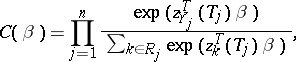(a1)

whereare the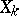ordered according to size,if it is itemthat fails at time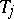, and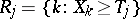denotes the set of itemsstill at risk, i.e. not yet failed, immediately before. With this setup, theth factor in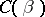describes the conditional distribution of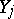given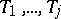and.

For many applications it is natural to allow for, e.g., censorings (cf. also Errors, theory of) or truncations (the removal of an item from observation through other causes than failure) as well as random covariate processes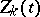. Formally this may be done by introducing the counting processes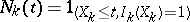registering the failures if they are observed, whereis a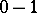-valued stochastic process with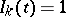if itemis at risk (under observation) just before time. If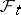denotes the-algebra for everything observed (failures, censorings, covariate values, etc.) on the time interval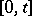, it is then required that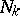have-intensity process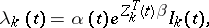(a2)

i.e.defines a-martingale (cf. also Martingale), while intuitively, for small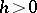, the conditional probability given the past that itemwill fail during the interval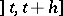is approximately, providedis at risk at time. Forknown, (a2) is then an example of Aalen's multiplicative intensity model [a1] with the integrated baseline hazard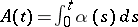estimated by, for any,(a3)

writing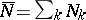and where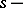signifies that it is the values ofand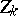just before the observed failure times that should be used. Since in practiceis unknown, in (a3) one of course has to replaceby the estimator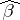, still obtained maximizing the partial likelihood (a1), replacingby the random number of observed failures, replacingby, and usingwithnow theth observed failure. (Note that in contrast to the situation with non-random covariates described above, there is no longer an interpretation of the factors inas conditional distributions.)

Using central limit theorems for martingales (cf. also Central limit theorem: Martingale), conditions may be given for consistency and asymptotic normality of the estimatorsand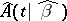, see [a3].

It is of particular interest to be able to test for the effect of one or more covariates, i.e. to test hypothesis of the formfor one or more given values of,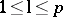. Such tests include likelihood-ratio tests derived from the partial likelihood (cf. also Likelihood-ratio test), or Wald test statistics based on the asymptotic normality of. A thorough discussion of the tests in particular and of the Cox regression model in general is contained in [a2], Sect. VII.2; [a2], Sect. VII.3, presents methods for checking the proportional hazards structure assumed in (a2).

Refinements of the model (a2) include models for handling e.g. stratified data, Markov chains with regression structures for the transition intensities, etc. It should be emphasized that these models, including (a2), are only partially specified in the sense that with (a2) alone nothing much is said about the distributions of theor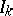. This, in particular, makes it extremely difficult to use the models for, e.g., the prediction of survival times.

How to Cite This Entry:
Cox regression model. Encyclopedia of Mathematics. URL: http://encyclopediaofmath.org/index.php?title=Cox_regression_model&oldid=16071
This article was adapted from an original article by Martin Jacobsen (originator), which appeared in Encyclopedia of Mathematics - ISBN 1402006098. See original article